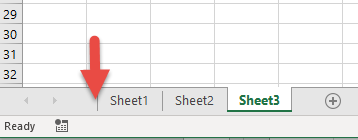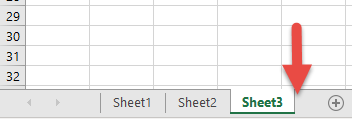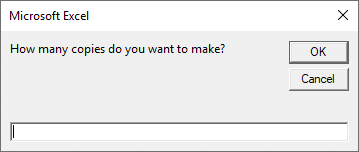# VBA Copy Worksheet

This tutorial will cover how to copy a Sheet or Worksheet using VBA.

## Copy Worksheet to New Workbook

To copy a Worksheet to a new Workbook:

``Sheets("Sheet1").Copy``

### Copy ActiveSheet to New Workbook

To copy the ActiveSheet to a new Workbook:

``ActiveSheet.Copy``

## Copy Multiple Sheets to New Workbook

To copy multiple Sheets to a new workbook:

``ActiveWindow.SelectedSheets.Copy``

## Copy Sheet Within Same Workbook

We started off by showing you the most simple copy Sheets example: copying Sheet(s) to a new Workbook.  These examples below will show you how to copy a Sheet within the same Workbook. When copying a Sheet within a Worbook, you must specify a location.  To specify a location, you will tell VBA to move the Worksheet BEFORE or AFTER another Worksheet.

### Copy Sheet Before Another Sheet

Here we will specify to copy and paste the Sheet before Sheet2

``Sheets("Sheet1").Copy Before:=Sheets("Sheet2")``

### Copy Sheet Before First Sheet

Instead of specifying the Sheet name, you can also specify the Sheet position. Here we are copying and pasting a Sheet before the first Sheet in the Workbook.

``Sheets("Sheet1").Copy Before:=Sheets(1)``

The newly created Sheet will now be the first Sheet in the Workbook.### Copy Sheet After Last Sheet

Use the After property to tell VBA to paste the Sheet AFTER another sheet. Here we will copy and paste a Sheet after the last Sheet in the Workbook:

``Sheets("Sheet1").Copy After:=Sheets(Sheets.Count)``

Notice that we used Sheets.Count to count the number of Sheets in the Workbook.## Move Sheet

You can also move a Sheet within a Workbook using similar syntax. This code will move Sheet1 to the end of the Workbook:

``Sheets("Sheet1").Move After:=Sheets(Sheets.Count)``

## Copy and Name Sheet

After copying and pasting a Sheet, the newly created sheet becomes the ActiveSheet. So to rename our new sheet, simply use ActiveSheet.Name:

``````Sub CopySheetRename1()

Sheets("Sheet1").Copy After:=Sheets(Sheets.Count)
ActiveSheet.Name = "LastSheet"

End Sub``````

If the Sheet name already exists, the above code will generate an error.  Instead we can use “On Error Resume Next” to tell VBA to ignore naming the Sheet and proceed with the rest of the procedure:

``````Sub CopySheetRename2()

Sheets("Sheet1").Copy After:=Sheets(Sheets.Count)
On Error Resume Next
ActiveSheet.Name = "LastSheet"
On Error GoTo 0

End Sub``````

Or use our RangeExists Function to test if the Sheet name already exists before attempting to copy the sheet:

``````Sub CopySheetRename3()

If RangeExists("LastSheet") Then
Else
Sheets("Sheet1").Copy After:=Sheets(Sheets.Count)
ActiveSheet.Name = "LastSheet"
End If

End Sub

Function RangeExists(WhatSheet As String, Optional ByVal WhatRange As String = "A1") As Boolean
Dim test As Range
On Error Resume Next
Set test = ActiveWorkbook.Sheets(WhatSheet).Range(WhatRange)
RangeExists = Err.Number = 0
On Error GoTo 0
End Function``````

VBA Programming | Code Generator does work for you!

### Copy and Name Sheet Based on Cell Value

You might also want to copy and name a Sheet based on a Cell Value.  This code will name the Worksheet based on the Cell value in A1

``````Sub CopySheetRenameFromCell()

Sheets("Sheet1").Copy After:=Sheets(Sheets.Count)
On Error Resume Next
ActiveSheet.Name = Range("A1").Value
On Error GoTo 0

End Sub``````

## Copy Worksheet to Another Workbook

So far we’ve worked with copying Sheets within a Workbook. Now we will cover examples to copy and paste Sheets to other Workbooks. This code will copy a Sheet to the beginning of another workbook:

``Sheets("Sheet1").Copy Before:=Workbooks("Example.xlsm").Sheets(1)``

This will copy a Worksheet to the end of another Workbook.

``Sheets("Sheet1").Copy After:=Workbooks("Example.xlsm").Sheets(Workbooks("Example.xlsm").Sheets.Count)``

Notice we replaced 1 with Workbooks(“Example.xlsm”).Sheets.Count to get the last Worksheet.

## Copy Worksheet to a Closed Workbook

You might also want to copy a Worksheet to a Workbook that is closed.  This code will open a closed Workbook so that you can copy a Sheet into it.

``````Sub CopySheetToClosedWB()
Application.ScreenUpdating = False

Set closedBook = Workbooks.Open("D:\Dropbox\excel\articles\example.xlsm")
Sheets("Sheet1").Copy Before:=closedBook.Sheets(1)
closedBook.Close SaveChanges:=True

Application.ScreenUpdating = True
End Sub``````

## Copy Sheet from Another Workbook Without Opening it

Conversely, this code will copy a Worksheet FROM a closed Workbook without you needing to manually open the workbook.

``````Sub CopySheetFromClosedWB()
Application.ScreenUpdating = False

Set closedBook = Workbooks.Open("D:\Dropbox\excel\articles\example.xlsm")
closedBook.Sheets("Sheet1").Copy Before:=ThisWorkbook.Sheets(1)
closedBook.Close SaveChanges:=False

Application.ScreenUpdating = True
End Sub``````

Notice that in both these examples we disabled ScreenUpdating so the process runs in the background.

## Duplicate Excel Sheet Multiple times

You can also duplicate an Excel Sheet multiple times by using a Loop.

``````Sub CopySheetMultipleTimes()
Dim n As Integer
Dim i As Integer
On Error Resume Next

n = InputBox("How many copies do you want to make?")

If n > 0 Then
For i = 1 To n
ActiveSheet.Copy After:=ActiveWorkbook.Sheets(Worksheets.Count)
Next
End If

End Sub``````